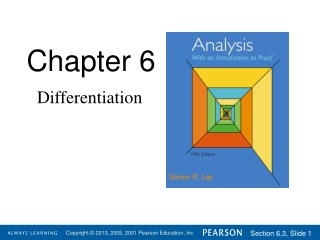DownloadDownload PresentationChapter 6

# Chapter 6

Télécharger la présentation## Chapter 6

- - - - - - - - - - - - - - - - - - - - - - - - - - - E N D - - - - - - - - - - - - - - - - - - - - - - - - - - -
##### Presentation Transcript

1. Chapter 6 Differentiation

2. Section 6.3 L’Hospital’s Rule

3. Consider the limit where f(x)  L and g(x)  M. If g(x)  0 for x close to c and M  0, then Theorem 5.1.13 says that If both L and M are zero, we can sometimes evaluate the limit by canceling a common factor in the quotient. In this section we derive another technique that is often easier to use than factoring and has wider application. In general, when L= M = 0, the limit of the quotient f /g is called an indeterminate form, because different values may be obtained for the limit. For example, for any real number k, let f(x) = kx and g(x) = x. Then So the indeterminate form (sometimes labeled 0/0) can lead to any real number as the limit.

4. Theorem 6.3.1 (Cauchy Mean Value Theorem) Letfand g be functions that are continuous on [a,b] and differentiable on (a,b). Then there exists at least one point c (a,b) such that [f(b) –f(a)]g(c)=[g(b) – g(a)]f(c). Proof: Let h(x)=[f(b)– f(a)]g(x) – [g(b)– g(a)] f(x) for each x[a,b]. Then h is continuous on [a,b] and differentiable on (a,b). Furthermore, h(a) =[f(b)– f(a)]g(a) – [g(b)– g(a)] f(a) = f(b)g(a) – g(b)f(a) h(b)= [f(b)– f(a)]g(b) – [g(b)– g(a)] f(b) = f(b)g(a) – g(b)f(a) Thus, by the mean value theorem (or Rolle’s Theorem), there exists c (a,b) such that h(c) = 0. So, h(a) = h(b). That is, [f(b) –f(a)]g(c) – [g(b) – g(a)]f(c)=0. 

5. In the expression [f(b) –f(a)]g(c)=[g(b) – g(a)]f(c), or, if g(x) = x for all x, then we have This formula is the original mean value theorem. Theorem 6.3.2 (L’Hospital’sRule) Let f and g be continuous on [a,b] and differentiable on (a,b). Suppose that c [a,b] and that f(c) = g(c) = 0. Suppose also that g(x)  0 for xU, where U is the intersection of (a,b) and some deleted neighborhood of c. If with L , then

6. We have c [a,b], f(c) = g(c) = 0, g(x)  0 for xU, and Let (xn) be a sequence in Uthat converges to c. Proof: Apply the Cauchy mean value theorem tofand g on the intervals [xn, c] or [c, xn] to obtain a sequence (cn) with cn between xn and c for each n, such that [f(xn) –f(c)]g(cn)=[g(xn)–g(c)]f(cn). Since g(x)  0 for all xU, and g(c) = 0, we must have g(xn)  0 for all n. (See Rolle’s Theorem.) Thus, since f(c) = g(c) = 0, we have L L for all n. Furthermore, since xnc and cn is between xn and c, it follows that cnc. Thus by Theorem 5.1.8, But then, so , also. 

7. Example 6.3.3 Then f(1) = g(1) = 0. Letf(x) = 2x2 – 3x + 1 and g(x) = x – 1. And we have f(x) = 4x – 3 and g(x) = 1, so that We did this same problem by factoring in Example 5.1.5. Example 6.3.4 Letf(x) = 1 – cosx and g(x) = x2. Then f(0) = g(0) = 0. And we have f(x) = sin x and g(x) = 2x, so that provided that the second limit exists. Since sin x0 and 2x0 as x0, we again have the indeterminate form 0/0. So we use l’Hospital’s rule again. Note that g(x) must be nonzero in a deleted neighborhood of 0, but it is permitted that g(0) = 0.

8. In some situations we wish to evaluate the limit of a function for larger and larger values of the variable. Definition 6.3.6 Let f: (a, )  . We say that the real number Lis the limit of fas x, and we write provided that for each > 0 there exists a real number N>a such that x>N implies that |f(x) – L| <. Very often as x the values of a given function also get large. This leads to the following definition. Definition 6.3.7 Let f: (a, ) . We say thatftends to  as x, and we write provided that given any there exists an N>a such thatx>N implies thatf(x) >. Using these definitions, we can state l’Hospital’s rule for indeterminates of the form /.

9. (L’Hospital’s Rule) Let f and g be differentiable on (a,). Suppose that limx f(x)=limxg(x)=, and that g(x)  0 for x (a,). If with L , then Theorem 6.3.8 The proof is lengthy, but basically consists of the Cauchy mean value theorem and algebra. There is also an extension of l’Hospital’s rule that applies to f/g when limx cf (x) = limx cg(x) =  and c  . (See Exercise 13.) There are other limiting situations involving two functions that can give rise to ambiguous values. These indeterminate forms are indicated by the symbols 0, 00, 1, 0, and  – , and are evaluated by using algebraic manipulations, logarithms, or exponentials to change them into one of the forms 0/0 or/.

10. Example 6.3.10 For x > 0, let f (x) = x and g(x) = – lnx. Then limx 0+ f (x)g(x) is an indeterminate of the form 0  . To evaluate the limit, we write  = 0.  From this we also conclude that limx 0+ xlnx = 0.